# Aspheric lenses for terahertz imaging

Jun. 15, 2022

We present novel designs for aspheric lenses used in terahertz (THz) imaging. As different surfaces result in different beam shaping properties and in different losses from reflection and absorption, the resultant imaging resolution (i.e. the focal spot size) depends critically on the design approach. We evaluate the different lens designs using Kirchhoff’s scalar diffraction theory, and test the predictions experimentally. We also show that our lenses can achieve sub-wavelength resolution. While our lens designs are tested with THz radiation, the design considerations are applicable also to other regions of the electro-magnetic spectrum.

## 1. Developments in the terahertz (THz) region

Over recent years there have been many new developments in the terahertz (THz) region. Techniques such as spectroscopy, package inspection, biological investigation etc advanced considerably. Most research is centered around high power emitters, quantum cascade lasers, and improving detection performance. However, little attention has been paid to improving imaging performance for THz imaging applications. Imaging of THz waves is usually achieved using off-axis parabolic mirrors (OAPMs) since spherical lenses are not appropriate because of the large beam diameters associated with THz radiation. Due to the much larger wavelength, the spatial resolution (which is directly related to the focal spot size) is quite limited. Moreover, if one wants to employ short focal lengths (~cm) to achieve small spot sizes, the wavelength becomes comparable to this length and near-field optics has to be considered.

The traditional approach of using OAPM for imaging is, in principle, diffraction limited. But OAPMs are susceptible to aberrations once misaligned, and the alignment is always difficult as the direction of the optical axis changes upon reflection off the mirror. Furthermore, the numerical aperture (NA) is limited as for high NAs the incident beam overlaps with the focal spot. Lenses, on the other hand, are much easier to align, less susceptible to aberrations, and allow higher NAs. Just placing the lenses on the optical axis with no tilt ensures proper alignment. While a parabolic surface is the only solution for a mirror to convert a plane wavefront into a spherical wavefront, a lens with its two surfaces allows an infinite number of solutions. Each solution will generate a different near-field pattern, and therefore the spatial resolution of the system will depend on the lens design. In this paper, we demonstrate different approaches to the lens design, and show that the resultant resolution can be sub-wavelength.

## 2. Lens design

The lens material we use is ultra high molecular weight p,olyethylene (UHMWPE) with a measured refractive index of 1.5245 and an absorption coefficient of 0.0135 mm -1. With its reasonably low absorption and negligible dispersion, UHMWPE is suitable as a lens material for even ultra-broadbandTHz radiation. Our lenses were milled on a computer-controlled lathe, resulting in a surface roughness of less than 30 µm (~λ/10). For all of the lenses studied here, we have chosen a focal length of 25 mm and a diameter of 50 mm, therefore the numerical aperture is 1 for all lenses (NA=d/(2f)). The focal length of our lenses is determined using the paraxial part of the beam.

### 2.1. Fermat’s principle

In order to simplify the calculations for the lens design, geometric optics is used to define both surfaces of the lens. It relies on Fermat’s principle where the entire wavefront from a collimated incident beam converges into a focal spot and each ‘ray’ travels the same optical distance. This ensures a lens design with no spherical aberration. It follows that when the focal length, the first surface, and the diameter of the lens are selected, there can be only one possible solution for the second surface. The second surface can be derived either analytically or numerically, depending on the shape of the first surface.

Geometric optics, however, does not provide an indication of the resultant focal spot size. In order to analyze the performance of our different lens designs, we use Kirchhoff’s scalar diffraction theory to determine the different focal spot sizes. This will be discussed in Section 4.

### 2.2. The planar-hyperbolic (p-h) lens

The most straight forward approach for an aberration-free aspheric lens is to set the first surface to be flat, with all refraction occuring at the second surface. Once the focal length of the lens has been fixed, this method has no degree of freedom except for the thickness of the lens. The resultant second surface is a hyperbola, hence the name of the lens. For this design the focal length is always equal to the distance from the tip of the lens (at the optical axis) to the focal spot. Figure 1(a) shows our planar-hyperbolic lens as used in the experiments.

It should be noted that the angle between the incident beam and the asymptote to the hyperbola is per definition the critical angle where total internal reflection starts to occur. For lenses with high NAs the beam will suffer large reflection losses.

### 2.3. The elliptical-aspheric (e-a) lens

In order to overcome the problem of the planar-hyperbolic lens, one can design a lens with the first surface to be curved as well. Choosing the first surface to be elliptical, we can derive an analytical solution for the second surface. This allows for more degrees of freedom when designing, namely the curvature of the first surface. An elliptical first surface will generate a spherical wavefront within the lens material that would generate a focus at a distance fe. The second surface images this spherical wavefront to another spherical wavefront behind the lens. Figure 1(b) shows our design for the elliptical-aspheric lens where we have chosen fe=202 mm.

### 2.4. The symmetric-pass (s-p) lens

While the e-a lens is able to partially solve the problem of the p-h lens, it is not trivial to optimize the reflection losses on both surfaces. As one would expect, that if each ray from the incident beam experiences the same angle of deviation on both surfaces while passing through the lens, the overall reflection loss would be minimum. This is similar to passing a beam through a prism at minimum deviation. Therefore we design our third lens solely based on this principle.

The symmetric-pass concept in a single lens or lens systems has been introduced previously for spherical lens surfaces, which proved to reduce spherical aberration considerably. However, they are only valid when paraxial approximation is applied. For high NAs these previous approaches lead to spherical aberration. Here we present for the first time a perfect symmetric-pass lens that that has no spherical aberration. There is, however, no analytical expression for both first and second surfaces. The entire lens profile is calculated numerically, starting from the edge of the lens. Figure 1(c) shows our symmetric-pass (s-p) lens.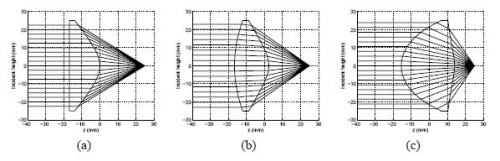Fig. 1. The three lenses we designed: (a) planar-hyperbolic lens; (b) elliptical-aspheric lens; (c) symmetric-pass lens.

## 3. Lens characteristics

### 3.1. General remarks

Although we have chosen the same diameter (50 mm) and the same focal length (25 mm) for all our lenses, they show different characteristics due to their different surface profiles. As all of the lenses produce spherical wavefronts, the only difference between the lenses is the amplitude distribution across the wavefront. The lens will affect this distribution in three different ways (see Fig. 2(a)): 1) reflection losses r 1(r) and r 2(r) at the two surfaces, 2) absorption losses α(r) within the lens, and 3) beam shaping δ(r) due to refraction. While the reflection and absorption losses should be taken into account, we found that they only play a minor role for determining the focal spot size. All our simulations were performed using a polarized Gaussian input beam with a FWHM of 25 mm at a frequency of 0.7 THz (corresponding to a wavelength of 0.43 mm). For such a beam the losses would reduce the beam power down to 75%, 74%, and 65% for the p-h, e-a and s-p lenses, respectively. The development of new THz materials might reduce these losses considerably.

### 3.2. Beam shaping, δ(r)

As we will show below, the focal spot size depends mainly on the beam shaping δ(r). It is obvious from Fig. 1 that for the different lens designs, the relation between the incident beam position and the angle it makes with the optics axis, the cone angle δ, is quite different. Figure 2(b) shows the δ(r) relations for our three lens designs.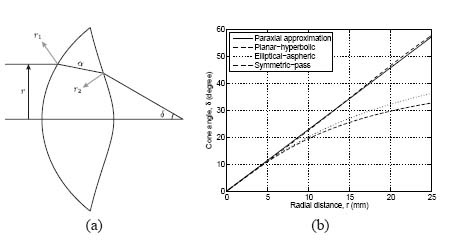Fig. 2. (a) Three different lens characteristics: 1) Reflection losses, r 1 and r 2; 2) Absorption losses, α(r); 3) Beam shaping, δ(r). (b) The δ(r) curve, showing the relation between the cone angle and the radial distance from the optics axis of the incident beam.

For a thin lens, where spherical surfaces are assumed and paraxial approximation is applied, the relation between δ(in radians) and r is simply linear. The gradient of the linear line is 1/f, where f is the focal length. For non-paraxial beams this relation becomes non-linear, and even for thin spherical lenses, spherical aberration occurs as well. It is clear from Fig. 2(b) that the p-h lens will give a narrow amplitude distribution after the lens, while the s-p will give the widest. As the focal plane is less than 100λ away from the lens, it is essential to use near-field theory to work out the precise intensity distribution in the focal plane.

## 4. Lens evaluations

### 4.1. Kirchhoff’s scalar diffraction theory

In order to determine the focal spot size, which is directly related to the spatial resolution of the imaging system, Kirchhoff’s scalar diffraction theory is used. Although derived from a different approach, it is based on the near-field Huygens-Fresnel principle. As an integral algorithm, it allows reasonably fast calculations with sufficient accuracy. Our algorithm is based on the following equation:

(1)

=∫()cos(−−2)EP=∫SK(θ)εArcos(kr−wt−π2)dS

where EP is the resultant electric field at point P as a result of all contributions from the incident wavefront; S is the input surface for the diffraction algorithm, in our case it is the second surface of the lens; εA is the amplitude of the input electric field on S; r is the distance between S and P; K(θ) is the obliquity factor. To speed up our simulations, we applied geometrical optics to trace the beam from the incident plane to the second surface of the lens taking into account the effects of reflection, absorption and refraction. The beam profile at the second lens surface is used as the input to the Kirchhoff’s scalar diffraction algorithm.

### 4.2. Simulation results

Since this is an integral calculation, we can chose the plane where we want to evaluate the output. While all the results we present here refer to the focal plane, we have calculated the output in other planes to ensure that the focus is at the position we expected. As the reflection losses are polarization dependent, the intensity profile in the focal plane is very slightly elliptical. Figure 3 shows the cross-sections of the intensity profiles of three different lenses on the axis that is perpendicular to the incident polarization. It can be clearly seen that the s-p lens not only gives the smallest FWHM but also has secondary diffraction maxima that are more than one order of magnitude lower than those for the p-h and e-a lenses. The secondary diffraction maxima arise due to the vignetting of the input beam at the lens but the magnitude of these maxima depend on the δ(r) relation. Table 1 shows the FWHM for central diffraction maximum for all three lenses and both polarizations using a frequency of 0.7 THz. We have also included simulations of loss-less lenses to indicate that the losses play only a minor role for determining the focal spot size. The numbers clearly show that the spot size using the s-p lens is more than 20% smaller compared with the other two lenses. Overall, our simulations predict that we should see a noticeable higher resolution when using the s-p lens but all three lenses produce a focal spot whose FWHM is smaller than one wavelength (0.44 mm). We have also evaluated the diffraction pattern in the focal plane of an OAPM with an effective focal length of 25 mm and NA=1, and the spot size is similar to the one of the s-p lens. But such an OAPM is not suitable for any imaging application as any extended sample in between two OAPMs would obstruct the incident and the outgoing beam.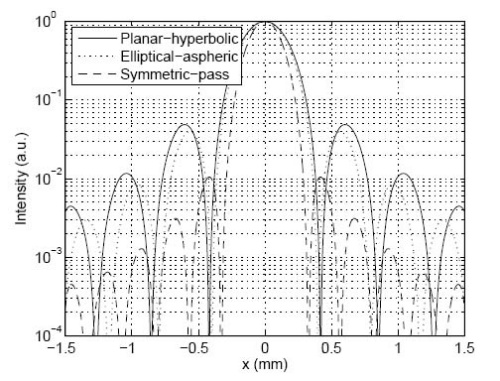Fig. 3. Intensity profiles of the focal spots from the different lenses, determined by Kirchhoff’s scalar diffraction theory and using a frequency of 0.7 THz.

Table 1. Focal spot sizes determined from Kirchhoff’s scalar diffraction theory.

 Lens Focal spot size, FWHM (mm) Parallel Perpendicular α=0 Planar-hyperbolic 0.372 0.383 0.378 Elliptical-aspheric 0.349 0.358 0.355 Symmetric-pass 0.269 0.279 0.276

## 5. Experiments

In order to verify the results from Kirchhoff’s scalar diffraction theory, we used a THz time domain spectroscopy (THz-TDS) experimental setup as shown in Fig. 4. The THz source is a surface emitter pumped by 80 fs, 800 nm pulses from a Ti:S laser, while the detector is a commercial THz antenna from EKSPLA which is gated by a time-delayed pulse from the same laser. The focal length of the OAPMs that were used to collimate and re-focus the THz beam was 75 mm. The current generated by the THz wave in the antenna was recorded with a lock-in amplifier, and the THz spectrum stretches from 0.1 THz to 1.5 THz with the peak at around 0.4 THz. Therefore we were able to evaluate the entire frequency range with a single scan of the time delay between pump and probe pulses. To evaluate the performance of a particular lens we inserted a pair of identical lenses into the THz path with the second lens facing the opposite direction.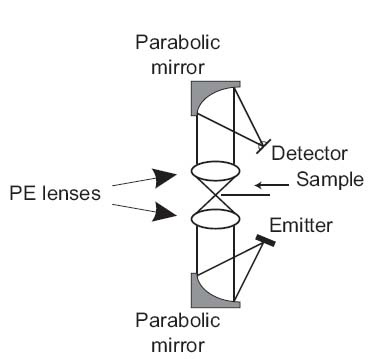Fig. 4. Experimental setup for measuring focal spot sizes.

First, we used a half-plane scan to determine the intensity distribution in the focal plane. For this purpose a razor blade was mounted as a ‘sample’ on the translation stage. It should be noted that, however, this method does not provide a perfect measurement of the focal spot sizes as we do not use a power meter with an active area large enough to capture the entire diffraction pattern. In our experimental setup other optical components after the focal plane limit the beam size imaged on the antenna which in turn doesn’t integrate over a large area. Nevertheless, as can be seen in Fig. 5, the measurement provides a very good indication of the focal spot size, and hence the spatial resolution for the imaging system. In the presentation of our results we have opted to only show the THz intensity at a frequency of 0.7 THz (using Fourier transformations) so that the experimental results can be compared more readily with our simulations. Figure 5 shows the experimental data for the three different lens pairs as well as the numerical integration using Eq. (1) across the part of the beam profile in the focal plane (see Fig. 3) that is not covered by the half-plane. There are no fitted parameters, only the position of the optical axis was adjusted and the power of the fully transmitted beam was set to unity. As the agreement between theory and experiment is exceptional, we can conclude that we have achieved sub-wavelengths resolution with the s-p lens producing the smallest spot size (see Table 1).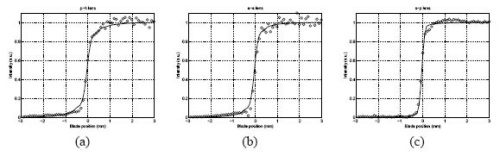Fig. 5. Half-plane scan results for the different lenses, at 0.7 THz: (a) Result for p-h lens; (b) Result for e-a lens; (c) Result for s-p lens.

In a second experiment we mounted a double pinhole (two holes in a 80µm-thick sheet of brass) as a sample. The holes have a diameter of 0.25 mm and are separated by 0.4 mm. Figure 6 shows a photo of the double pinhole and the THz image measured by performing x-y scans (with a step size of 50 µm in each direction) in the focal planes of the three different lenses. Here we show the THz intensity integrated over the entire spectral range with a central wavelength of 0.4 THz (λ=0.75 mm).

The experiments clearly show that the s-p lens pair (Fig. 6(d)) gives by far the best resolution with an intensity at the saddle point between the two primary diffraction maxima of 30.8%. The values for the p-h (Fig. 6(b)) and the e-a (Fig. 6(c)) lens pairs are 88.4% and 84.8%, respectively. According to Rayleigh’s criterion the images of the two pin holes are resolved if the intensity at the saddle point is less than 81%, and therefore only the s-p lens pair clearly resolves the two pinholes. Using only the frequency component at 0.7 THz (λ=0.43 mm) the values for the saddle points are: (b) 86.8%, (c) 73.1%, and (d) 14.4%. Here both the e-a and the s-p lenses resolve the image but again the s-p lenses perform far better. As the separation between the two pinholes is 0.4 mm, this experiment conclusively demonstrates that we can achieve sub-wavelength resolution with the s-p lens pair.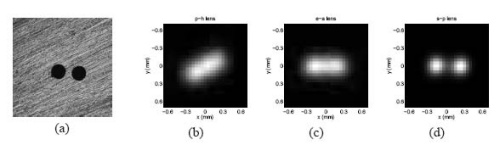Fig. 6. (a) Double pinhole used as the imaging sample; (b) Result for p-h lens; (c) Result for e-a lens; (d) Result for s-p lens.

## 6. Conclusion

We have presented novel designs for aspheric THz lenses, and have shown both numerically and experimentally that sub-wavelength resolution can be achieved with such lenses. While we have chosen a fixed focal length of f=25 mm for our investigations, the lens designs can be applied to another focal length as well. The spot size in the focal plane was calculated using Kirchhoff’s scalar diffraction theory, and the results were confirmed using a THz-TDS setup. The symmetric-pass lens performed by far the best, resulting in focal spot size (FWHM) of about 0.3 mm at a wavelength of 0.44 mm, and very clearly resolving two pinholes that are separated by 0.53λ. While our current lenses are made out of UHMWPE and therefore have considerable losses, using other THz materials should alleviate this problem. Compared to OAPMs, our aspheric lenses can have a much larger NA and are easier to align. As all the parameters can be scaled with the wavelength, our novel designs are also applicable to other regions of the electro-magnetic spectrum.